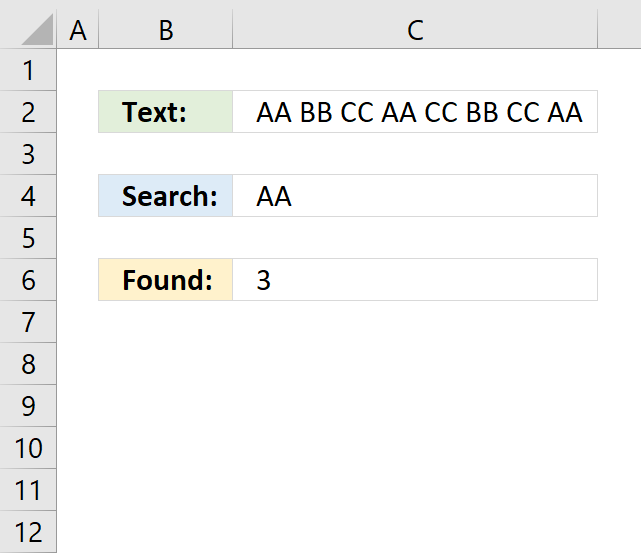Author: Oscar Cronquist Article last updated on October 15, 2018

Question: How do I count how many times a text string exists in a cell value in Excel?The formula in cell C6 counts how many times a given text string is found in a cell value.

Formula in C6:

=(LEN(C2)-LEN(SUBSTITUTE(C2, C4, "")))/LEN(C4)

### Explaining formula in cell C6

#### Step 1 - Substitute given text string with nothing

SUBSTITUTE(C2, C4, "")

becomes

SUBSTITUTE("AA BB CC AA CC BB CC AA", "AA", "")

and returns " BB CC CC BB CC ".

#### Step 2 - Count text string characters

LEN(SUBSTITUTE(C2, C4, ""))

becomes

LEN(" BB CC CC BB CC ")

and returns 17.

#### Step 3 - Count text string characters in cell C2

LEN(C2)

becomes

LEN("AA BB CC AA CC BB CC AA")

and returns 23.

#### Step 4 - Subtract original character length with new text string character length

LEN(C2)-LEN(SUBSTITUTE(C2, C4, ""))

becomes

23 - 17

and returns 6.

#### Step 5 - Divide with search string character length

(LEN(C2)-LEN(SUBSTITUTE(C2, C4, "")))/LEN(C4)

becomes

6/LEN(C4)

becomes

6/2

and returns 3 in cell C6.